# CHAR Function

Returns a character specified by a number

## What is the CHAR Function?

The CHAR function is categorized under Excel Text Functions. It returns a character specified by a number. As a financial analyst, the CHAR function is useful in coding page numbers received from other files into characters, or when we wish to insert a line break into text.

### Formula

=CHAR(number)

The CHAR function uses the following argument:

1. Number (required argument) – This is a number between 1 and 255 specifying which character we want. The character is from the character set used by our computer.

The character set may vary across different operating systems and so, for a specific number, the function may return different results on different computers.

Windows OS uses the ANSI character set, whereas Macintosh uses the Macintosh character set.

### How to use CHAR Function in Excel?

The CHAR function is a built-in function and can be used as a worksheet function in Excel. As a worksheet function, the CHAR function can be entered as part of a formula in a cell of a worksheet.

To understand the uses of this function, let’s consider few examples:

#### Example 1

Let’s see the results we get we use the CHAR function on the data below: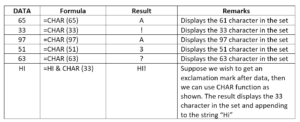The result in Excel is shown below: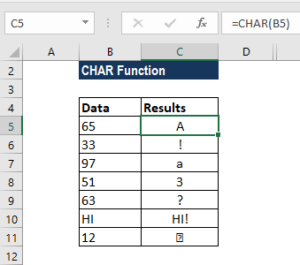If we are using this function by providing a reference to a cell, then it should not be in inverted commas.  The above results are from the ANSI character set (used on the Windows operating system).

#### Example 2

As mentioned above, one of the uses of the CHAR function is inserting line breaks into text. To understand this, let’s take an example: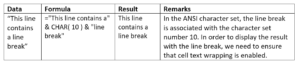The results in Excel are shown below: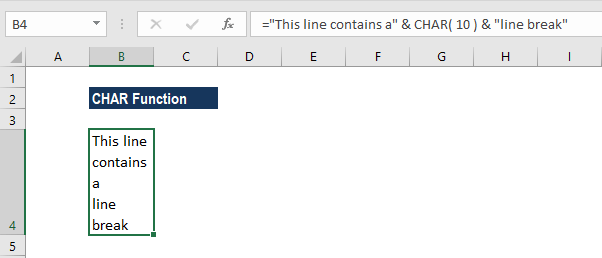To enable text wrapping, we can use the Wrap Text button, which is located in the ‘Alignment’ group in the Home tab of the Excel ribbon, as shown below: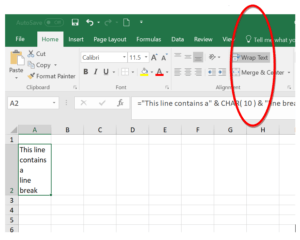### A few things to remember about the CHAR function:

1. VALUE# error – It occurs when:
a. The given argument is not an integer between 1 to 255.
b. If the argument entered is zero.

2. The function will give the result as a string/text value.

3. It was introduced in Excel 2000 version and is available in all versions after that.

4. The CHAR function is the inverse of the CODE function.

5. Excel functions CHAR and UNICHAR will return the character for a given code whereas Excel functions CODE and UNICODE would do the opposite – give the code for a given character.

6. It is also available in VBA.

7. For numbers greater than 255, we can use the UNICHAR function.

Thanks for reading CFI’s guide to important Excel functions! By taking the time to learn and master these functions, you’ll significantly speed up your financial analysis. To learn more, check out these additional CFI resources:

• Excel Functions for Finance